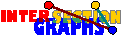# Some NP-completeness results

What if recognition is NP-complete? How could we prove it? Remember the splitting of the recognition problem in the dualization approach. If we could prove the hardness of the first step, i.e. the hardness of finding some list of graphs which could serve as star graphs, then the reconstruction problem is hard too, since every representation immediately yields star graphs. There might be cases, however, where we still don`t know anything about the complexity of the of the recognition problem if the first step is hard. There might be other ways to recognize the graphs in question.

In general, hardness of the second subproblem doesn`t imply hardness of the reconstruction of recognition problems, since the instances delivered by the first step might be tamer than expected.

Therefore, NP-completeness results should be easier to achieve in discrete models, where the second step, the layout step, is mostly trivial. This is indeed the case. I know of only two NP-completeness result for recognition of geometrically defined intersection graphs, namely boxes in Rd, where d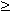3, and unit-disk graphs [BK98]. Roughly speaking, for most geometric models, the intersection graphs seem difficult to recognize but to prove the hardness of the problem seems also difficult.

Let us now treat some discrete models where in the first step of the approach it is NP-complete to decide whether suitable star graphs exist, but where step 2, expressed by some Krausz-type characterization, is trivial. Then recognition, as well as reconstruction, are NP-hard.

Let(G) denote the smallest integer for which a graph G is the intersection graph of some (not necessarily simple) k-uniform hypergraph.[PRT81]: Deciding whether(G)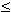k for instances G and k is NP-complete.
 Proof: We reduce from the chromatic number problem. Let n be the number of vertices of G. We define H=K1*(nK1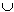G), i.e. H is obtained by adding new vertices y1,y2,..., yn,x to G and joining x to all vertices of V(G){y1,...,yn}. Using the Krausz-type characterization one can show that the chromatic number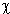(G) of the complement --G of G (i.e. the clique covering number of G, the minimum number of cliques necessary to cover all vertices of G) equals(H) -n.Canbe approximated?

Still the reduction doesn't say anything about the complexity of the problem(G)k for k|V(G)|/2 +2, for instance. For fixed k=3 and simple hypergraphs we use a reduction from 3-SAT, variants of which seem to work in several of these situations.[PRT81]: Recognizing graphs in G0(3-element sets) is NP-complete.

Proof: Let c1 & ... & cm be an instance of 3-SAT, a boolean expression in variables x,y,z,.... We may assume that every clause contains exactly 3 distinct literals.

For every variable x we construct a graph Hx as shown to the right. Some of its edges are labelled x1,..., xm, ¬x1,... , ¬xm.For every clause ci we construct a graph Fi, where three edges are labeled by the literals occuring in ci. Now we take the disjoint union of all graphs and glue together edges with identical labels (in an arbitrary orientation), see below.C1=x and y and z C2= ¬x and y and w C3=x and ¬z and ¬w C4=x and ¬y and ¬w

Now those coverings obeying the condition mentioned in the Krausz-type characterization correspond 1-1 to those assignments of values to the variables x,y,z, ... where c1 & ... & cm is true.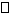Although a similiar reduction shows that recognizing members of G(4-element sets) is also NP-complete, the complexity of recognizing members of G(3-element sets) (without requiring simplicity of the hypergraph, that is) is still unsettled.

For intersection graphs of 3-uniform linear hypergraphs there is a similiar reduction, showing that recognizing intersection graphs of linear 3-uniform hypergraphs is NP-complete [HK97]. The construction also shows that even for graphs of minimum degree at least 4 the problem is hard.

Back to the start page for intersection graphs.

Erich Prisner